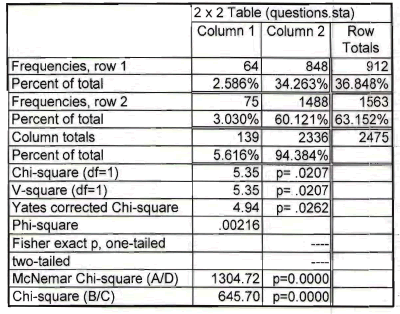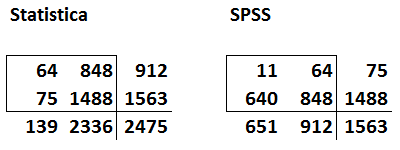# Discrepancies in the chisquare test

## 2011-12-16

This page is currently being updated from the earlier version of my website. Sorry that it is not yet fully available.

I was working with two researchers on a project and they got different results for their chisquare tests. See if you can find out what went wrong.

Here’s the output from Statistica.The chisquare statistics is 5.35 and the p-value is 0.0207.

Here’s the output from SPSS.The chisquare statistic is 23.603 and the p-value is 0.000. Now obviously the discrepancy occurs because the numbers in the table are different, but why did tow people working with the same data set enter different data into their programs? Can you figure it out?

If you need a hint, here’s the table from the first and second tables shown side by side.Have you figured it out yet?

Look for common numbers in the tables. Notice that the first row of data in Statistica is the same as the second column of data in SPSS.

Side by side table with common numbers highlighted

Also notice that the second row of data in Statistica is the third column (the totals) for the data in SPSS.So the problem is that the data input into Statistica represents the number with a particular outcome (row 1) and the total (row 2). You need to make one of the rows the number with a particular outcome and the other the number without the particular outcome. Otherwise, you end up double counting some of the data.

The other tip-off as to which table is correct is the grand total. The true sample size in this problem is 1,563 which matches the number in the lower right hand corner of the SPSS table. The data is entered into Statistica, has an incorrect grand total of 2,475 because some patients contribute to the counts in both the first and the second rows in the Statistica table.

It’s an easy mistake to make. When you are entering data into a two by two table, be sure that a patient can belong to only one of the four cells in the table. If a patient can potentially contribute to the count in more than one cell, then you have artificially inflated your sample size and your chisquare statistic will be invalid.

You can find an earlier version of this page on my original website.